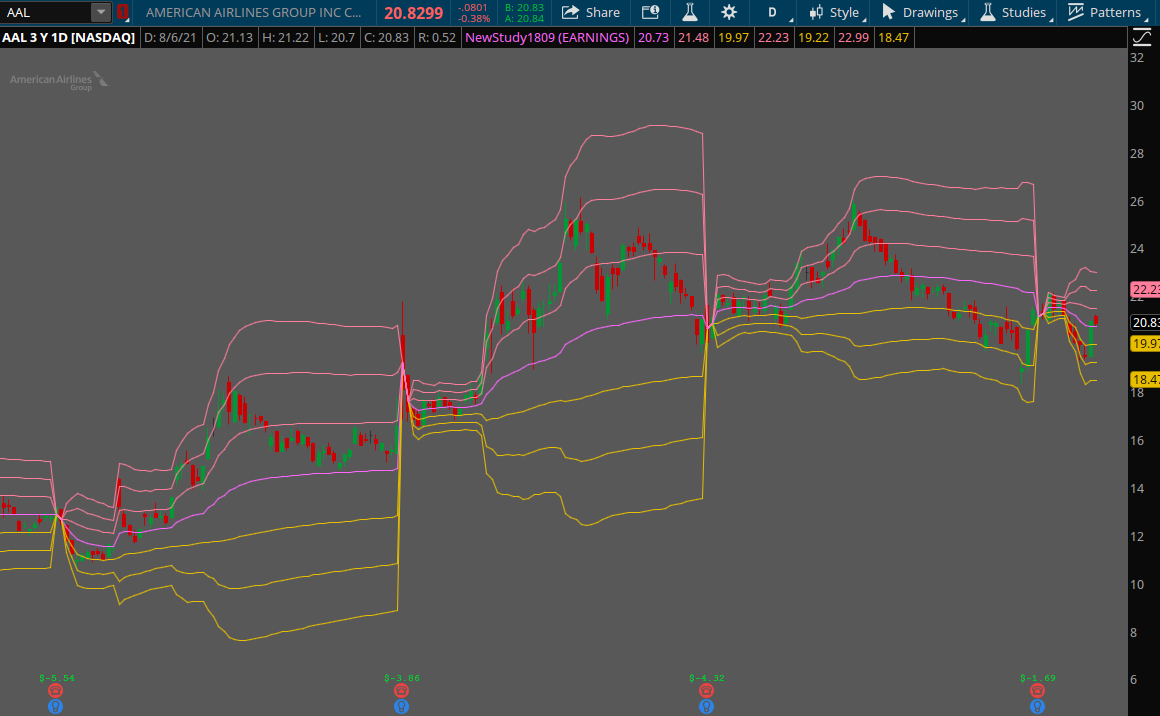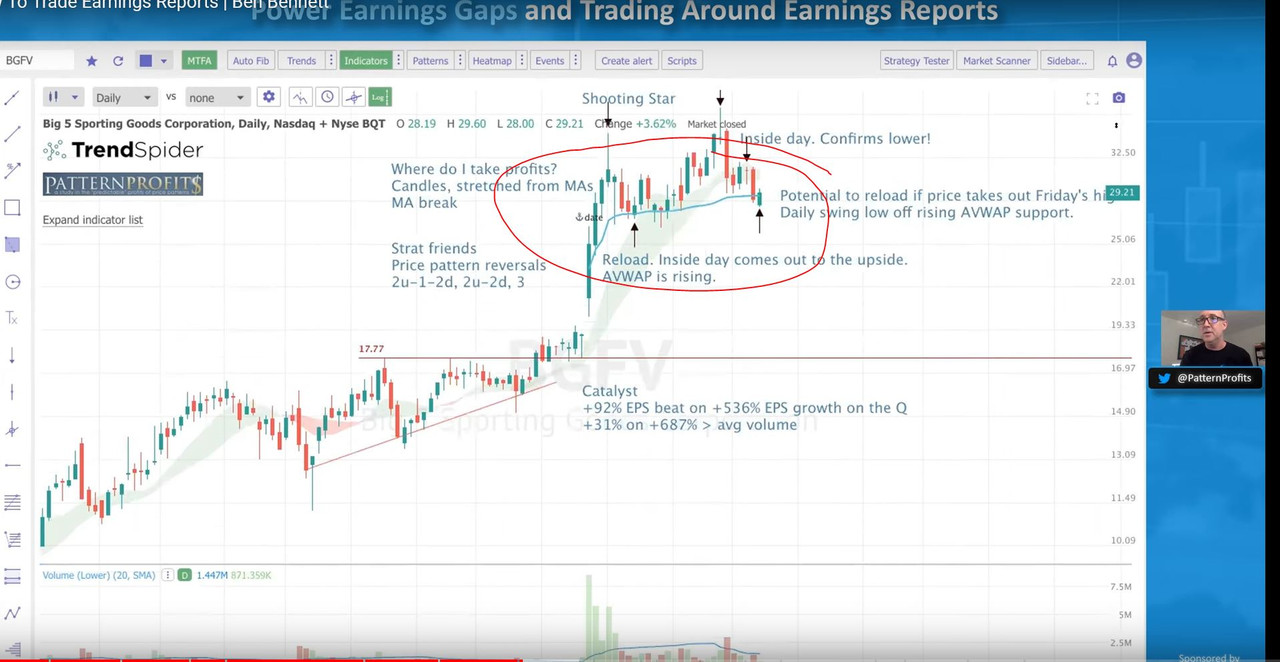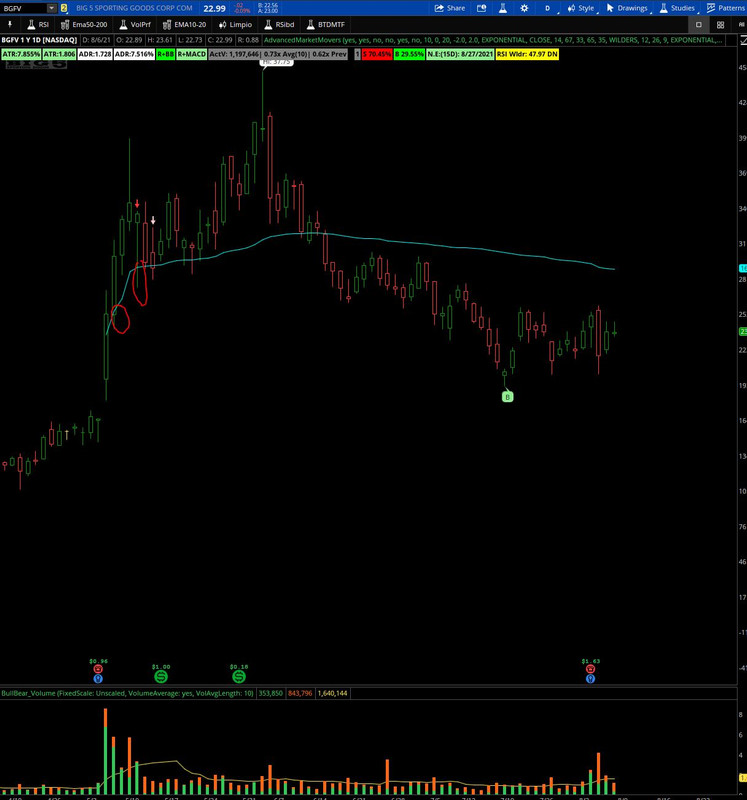# Anchored VWAP Indicator for ThinkorSwim

#### SleepyZ

##### Well-known member
Hello, @SleepyZ I am happy to report that the script works nicely. It does seem to reset itself from Monday (D1) and etc, so on Monday the 2D and 3D lines do not show up unless I set N as 3 on Monday. Is there a way to automatically rolling back for the 3 days? It seems to roll automatically on futures though. Many thanks!
This is one way to fix the above issue when non-trading days are included in the last three days:
Code:
``````#VWAP Anchored
#20210522 Sleepyz - Usethinkscript request
#20210525 Sleepyz - modified to handle when no trading days are involved within the last 3 days

script v {
input lookback = 1;
def ymd      = GetYYYYMMDD();
def dayCount = CompoundValue(1, if ymd != ymd then dayCount + 1 else dayCount, 0);
def thisDay  = (HighestAll(dayCount) - dayCount) ;
def anchor   =  1 + lookback ;
def volumeSum      = if thisDay <= anchor
then CompoundValue(1, volumeSum + volume, volume)
else 0;
def volumeVwapSum  = if thisDay <= anchor
then CompoundValue(1, volumeVwapSum + volume * vwap, volume * vwap)
else 0;
def volumeVwap2Sum = if thisDay <= anchor
then CompoundValue(1, volumeVwap2Sum + volume * Sqr(vwap),
volume * Sqr(vwap))
else 0;
def price     = volumeVwapSum / volumeSum;
def deviation = Sqrt(Max(volumeVwap2Sum / volumeSum - Sqr(price), 0));
plot VWAP     = price;
}
#Day Separator
input show_day_separator = yes;
AddVerticalLine(show_day_separator and SecondsFromTime(0930) == 0, "", Color.BLUE, stroke = Curve.FIRM);

input lookback = 0;
plot v1 = v(lookback);
plot v2 = v(lookback + 1);
plot v3 = v(lookback + 2);
v1.SetDefaultColor(Color.CYAN);
v2.SetDefaultColor(Color.GREEN);
v3.SetDefaultColor(Color.RED);
v1.SetLineWeight(3);
v2.SetLineWeight(3);
v3.SetLineWeight(3);
v3.SetStyle(Curve.LONG_DASH);``````
Here is an ONDEMAND chart with the current day a Monday displaying the Monday's developing vwap and the prior two trading days•Jonas99

##### New member
Do you all have a colored vwap script? Vwap that will be red if price is under and green if price is above?

#### MerryDay

Staff member
Staff
VIP
•#### alexsilva_ni

##### New member
hey,

I'm trying to automate the anchored vwap to take the lowest date of my daily chart with the following code, but somehow Thinkorswim it is not respecting the date that comes from "GetValue(GetYYYYMMDD(), GetMinValueOffset(low, 145));". If I hardcode the date like: def anchorDate = 20210325; it works. does anybody know what I'm missing, thanks for any help, regards,

def anchorDate = GetValue(GetYYYYMMDD(), GetMinValueOffset(low, 145));

def todayDate = GetYYYYMMDD();

def postAnchorDate = if todayDate >= anchorDate then 1 else 0;

plot anchoredVWAP = TotalSum(if postAnchorDate then ((high + low + close) / 3) * (volume) else 0) / TotalSum(if postAnchorDate then volume else 0);

anchoredVWAP.SetStyle(Curve.FIRM);
anchoredVWAP.SetLineWeight(3);
anchoredVWAP.SetDefaultColor(Color.CYAN);

#### SleepyZ

##### Well-known member
hey,

I'm trying to automate the anchored vwap to take the lowest date of my daily chart with the following code, but somehow Thinkorswim it is not respecting the date that comes from "GetValue(GetYYYYMMDD(), GetMinValueOffset(low, 145));". If I hardcode the date like: def anchorDate = 20210325; it works. does anybody know what I'm missing, thanks for any help, regards,

def anchorDate = GetValue(GetYYYYMMDD(), GetMinValueOffset(low, 145));

def todayDate = GetYYYYMMDD();

def postAnchorDate = if todayDate >= anchorDate then 1 else 0;

plot anchoredVWAP = TotalSum(if postAnchorDate then ((high + low + close) / 3) * (volume) else 0) / TotalSum(if postAnchorDate then volume else 0);

anchoredVWAP.SetStyle(Curve.FIRM);
anchoredVWAP.SetLineWeight(3);
anchoredVWAP.SetDefaultColor(Color.CYAN);

Change this line and see if it works as you expect

Code:
``def anchorDate = highestall(GetValue(GetYYYYMMDD(), GetMinValueOffset(low, 145)));``

#### Ahmar824

##### Member
hey,

I'm trying to automate the anchored vwap to take the lowest date of my daily chart with the following code, but somehow Thinkorswim it is not respecting the date that comes from "GetValue(GetYYYYMMDD(), GetMinValueOffset(low, 145));". If I hardcode the date like: def anchorDate = 20210325; it works. does anybody know what I'm missing, thanks for any help, regards,

def anchorDate = GetValue(GetYYYYMMDD(), GetMinValueOffset(low, 145));

def todayDate = GetYYYYMMDD();

def postAnchorDate = if todayDate >= anchorDate then 1 else 0;

plot anchoredVWAP = TotalSum(if postAnchorDate then ((high + low + close) / 3) * (volume) else 0) / TotalSum(if postAnchorDate then volume else 0);

anchoredVWAP.SetStyle(Curve.FIRM);
anchoredVWAP.SetLineWeight(3);
anchoredVWAP.SetDefaultColor(Color.CYAN);

Can you repost the new code with the automatic date change thank you

#### alexsilva_ni

##### New member
Can you repost the new code with the automatic date change thank you

sure, code below:

def anchorDate = highestall(GetValue(GetYYYYMMDD(), GetMinValueOffset(low, 145)));

def todayDate = GetYYYYMMDD();

def postAnchorDate = if todayDate >= anchorDate then 1 else 0;

plot anchoredVWAP = TotalSum(if postAnchorDate then ((high + low + close) / 3) * (volume) else 0) / TotalSum(if postAnchorDate then volume else 0);

anchoredVWAP.SetStyle(Curve.FIRM);
anchoredVWAP.SetLineWeight(3);
anchoredVWAP.SetDefaultColor(Color.CYAN);

•Ahmar824

#### torewy

##### New member
I'm basically trying to figure out how to modify the previously shared code below so that it shows market-hours-only VWAP on all days, not just today. Many thanks. - Tom

def anchorTime = 0930;
def anchorEnd = 1600;

input ShowTodayOnly = yes;
def Today = if GetDay() == GetLastDay() then 1 else 0;
def postAnchorTime = if SecondsFromTime(anchorTime) >= 0 then 1 else 0;
def endAchorTime = if SecondsTillTime(anchorEnd) >= 0 then 1 else 0;

def volumeSum = compoundValue(1, if postAnchorTime and endAchorTime then volumeSum + volume else 0, volume);
def volumeVwapSum = compoundValue(1, if postAnchorTime and endAchorTime then volumeVwapSum + volume * vwap else 0, volume * vwap);

plot anchorVWAP = if ShowTodayOnly and !Today then Double.NaN else if anchorTime then volumeVwapSum / volumeSum else Double.NaN;
anchorVWAP.setStyle(Curve.Firm);
anchorVWAP.setDefaultColor(Color.light_ORANGE);
anchorVWAP.setlineWeight(2);

#### SleepyZ

##### Well-known member
I'm basically trying to figure out how to modify the previously shared code below so that it shows market-hours-only VWAP on all days, not just today. Many thanks. - Tom

def anchorTime = 0930;
def anchorEnd = 1600;

input ShowTodayOnly = yes;
def Today = if GetDay() == GetLastDay() then 1 else 0;
def postAnchorTime = if SecondsFromTime(anchorTime) >= 0 then 1 else 0;
def endAchorTime = if SecondsTillTime(anchorEnd) >= 0 then 1 else 0;

def volumeSum = compoundValue(1, if postAnchorTime and endAchorTime then volumeSum + volume else 0, volume);
def volumeVwapSum = compoundValue(1, if postAnchorTime and endAchorTime then volumeVwapSum + volume * vwap else 0, volume * vwap);

plot anchorVWAP = if ShowTodayOnly and !Today then Double.NaN else if anchorTime then volumeVwapSum / volumeSum else Double.NaN;
anchorVWAP.setStyle(Curve.Firm);
anchorVWAP.setDefaultColor(Color.light_ORANGE);
anchorVWAP.setlineWeight(2);#### whoof

##### New member
Loving this indicator but when I set a starting date, how do I have it only stick for the current symbol that I'm looking at? For example if I pick out a high manually on AAPL, it wouldn't make sense to carry over the exact indicator settings to another ticker when I change my chart view

#### SleepyZ

##### Well-known member
Loving this indicator but when I set a starting date, how do I have it only stick for the current symbol that I'm looking at? For example if I pick out a high manually on AAPL, it wouldn't make sense to carry over the exact indicator settings to another ticker when I change my chart view

Enter the symbol you want to limit the indicator at the 'input symbol' in the following code

Code:
``````input symbol     = "AAPL";
input anchorDate = 20200422;
input anchorTime = 2030;

def inPeriod = if SecondsTillTime(tradeStartEST) <= 0 and SecondsTillTime(tradeEndEST) > 0 then 1 else 0;

def revisedDate = if SecondsTillTime(anchorTime)<=0 and !inPeriod then anchorDate+1 else if SecondsTillTime(anchorTime)<=0 and inPeriod then anchorDate else anchorDate;

def postAnchorDate = if GetYYYYMMDD() >= revisedDate then 1 else 0;
def postAnchorTime = if SecondsTillTime(anchorTime) <= 0 then 1 else 0;

plot anchoredVWAP = if getsymbol()!=symbol then double.nan else TotalSum(if postAnchorDate and postAnchorTime then ((high+low+close)/3)*(volume) else 0)/TotalSum(if postAnchorDate and postAnchorTime then volume else 0);

anchoredVWAP.setStyle(Curve.Firm);
anchoredVWAP.SetLineWeight(3);
anchoredVWAP.SetDefaultColor(Color.Cyan);

•Kitchasap

#### whoof

##### New member
Enter the symbol you want to limit the indicator at the 'input symbol' in the following code
Thank you. This is interesting. Is there a way to have a list? That way I can have say 2 AVWAPs on AAPL and 1 on goog? I like that tradingview and tc2000 stick these to the symbol so you can always reference it again when you step back down the line

#### SleepyZ

##### Well-known member
Thank you. This is interesting. Is there a way to have a list? That way I can have say 2 AVWAPs on AAPL and 1 on goog? I like that tradingview and tc2000 stick these to the symbol so you can always reference it again when you step back down the line
You can load multiple copies of the script and change the symbols at the input. The scripts will only display the one associated with the chart symbol

#### esti0000

##### New member
VIP
I have an anchored VWAP code with standard deviations and I was wondering if anyone could modify it to automatically anchor to the day of the last earnings report. code is below thanks

Code:
``````# input is bar 126 bc that is the last bar on the 6M 1D Timeframe I use
input Number_Of_Bar1 = 126;
#I set it to 14 days back as that seems to be norm with other indicators like RSI
def Number_Of_Bar = Number_of_Bar1 - 14;
def Data = BarNumber();
input price = ohlc4;
def bar =  Data >= Number_Of_Bar;
def pv = if bar then pv + price * volume else 0;
def cumvolume = if bar then cumvolume + volume else 0;
plot vw = pv / cumvolume;
def bars = data - number_Of_Bar;
def sample = if bar then sample + sqr(price - vw) else 0;
def var = sample/bars;
def dev = sqrt(var);
plot dev1 =  vw + dev;
plot dev2 = vw + (dev * 2);
plot dev3 = vw + (dev * 3);
plot devN1 =  vw - dev;
plot devN2 = vw - (dev * 2);
plot devN3 = vw - (dev * 3);``````
Was wondering if anyone has been able to provide a solution for this? Anchor VWAP automatically to last earnings date?

#### SleepyZ

##### Well-known member
Was wondering if anyone has been able to provide a solution for this? Anchor VWAP automatically to last earnings date?
Here is the standard TOS vwap with an Earnings option added.Ruby:
``````#
# TD Ameritrade IP Company, Inc. (c) 2011-2021
#

input timeFrame = {default DAY, WEEK, MONTH, EARNINGS};

def cap = GetAggregationPeriod();
def errorInAggregation =
timeFrame == timeFrame.DAY and cap >= AggregationPeriod.WEEK or
timeFrame == timeFrame.WEEK and cap >= AggregationPeriod.MONTH;
Assert(!errorInAggregation, "timeFrame should be not less than current chart aggregation period");

def hearn  = if HasEarnings() then GetYYYYMMDD() else Double.NaN;
def yyyyMmDd = GetYYYYMMDD();
def periodIndx;
switch (timeFrame) {
case DAY:
periodIndx = yyyyMmDd;
case WEEK:
periodIndx = Floor((DaysFromDate(First(yyyyMmDd)) + GetDayOfWeek(First(yyyyMmDd))) / 7);
case MONTH:
periodIndx = RoundDown(yyyyMmDd / 100, 0);
case EARNINGS:
periodIndx = !IsNaN(hearn);
}
def isPeriodRolled = CompoundValue(1, periodIndx != periodIndx, yes);

def volumeSum;
def volumeVwapSum;
def volumeVwap2Sum;

if (isPeriodRolled) {
volumeSum = volume;
volumeVwapSum = volume * vwap;
volumeVwap2Sum = volume * Sqr(vwap);
} else {
volumeSum = CompoundValue(1, volumeSum + volume, volume);
volumeVwapSum = CompoundValue(1, volumeVwapSum + volume * vwap, volume * vwap);
volumeVwap2Sum = CompoundValue(1, volumeVwap2Sum + volume * Sqr(vwap), volume * Sqr(vwap));
}
def price = volumeVwapSum / volumeSum;
def deviation = Sqrt(Max(volumeVwap2Sum / volumeSum - Sqr(price), 0));

plot VWAP = price;
plot UpperBand = price + 1 * deviation;
plot LowerBand = price - 1 * deviation;

VWAP.SetDefaultColor(GetColor(0));
UpperBand.SetDefaultColor(GetColor(2));
LowerBand.SetDefaultColor(GetColor(4));

plot UpperBand2 = price + 2 * deviation;
plot LowerBand2 = price - 2 * deviation;

UpperBand2.SetDefaultColor(GetColor(2));
LowerBand2.SetDefaultColor(GetColor(4));

plot UpperBand3 = price + 3 * deviation;
plot LowerBand3 = price - 3 * deviation;

UpperBand3.SetDefaultColor(GetColor(2));
LowerBand3.SetDefaultColor(GetColor(4));``````

•esti0000 and MerryDay

#### Xhrx

##### New member
VIP

Code:
``````Code:
input anchorDate = 20200422;
input anchorTime = 2030;

def inPeriod = if SecondsTillTime(tradeStartEST) <= 0 and SecondsTillTime(tradeEndEST) > 0 then 1 else 0;

def revisedDate = if SecondsTillTime(anchorTime)<=0 and !inPeriod then anchorDate+1 else if SecondsTillTime(anchorTime)<=0 and inPeriod then anchorDate else anchorDate;

def postAnchorDate = if GetYYYYMMDD() >= revisedDate then 1 else 0;
def postAnchorTime = if SecondsTillTime(anchorTime) <= 0 then 1 else 0;

plot anchoredVWAP = TotalSum(if postAnchorDate and postAnchorTime then ((high+low+close)/3)*(volume) else 0)/TotalSum(if postAnchorDate and postAnchorTime then volume else 0);

anchoredVWAP.setStyle(Curve.Firm);
anchoredVWAP.SetLineWeight(3);
anchoredVWAP.SetDefaultColor(Color.Cyan);

Do I need to change the anchorTime to 09:30am as the day starts at that point or I leave it on 20:30?

#### MerryDay

Staff member
Staff
VIP
@Xhrx The script you posted is the ADJUSTABLE TIME VERSION. You can change the time to whatever suits your strategy. The 2nd script in post#1 is the intraday day version that starts at 09:30. Use whatever fits your trading. You can plug&play the many flavors of this indicator found in this thread to get a better understanding and to modify the inputs to fit your trading.

•QUIKTDR1

#### Xhrx

##### New member
VIP
Thanks @MerryDay for the explanation. I have found a difference between Trendspider Anchored Vwap and this indicator

and look the ImageAnd I tried to do the same on ThinkOrswim but I've found some slightly difference. I'm not sure why there's a difference on anchored Vwap (It's a minor difference)•QUIKTDR1

##### New member
Does anyone have a Thinkorswim scan for when the stock price crosses over the Anchored VWAP? Not vwap but AVWAP